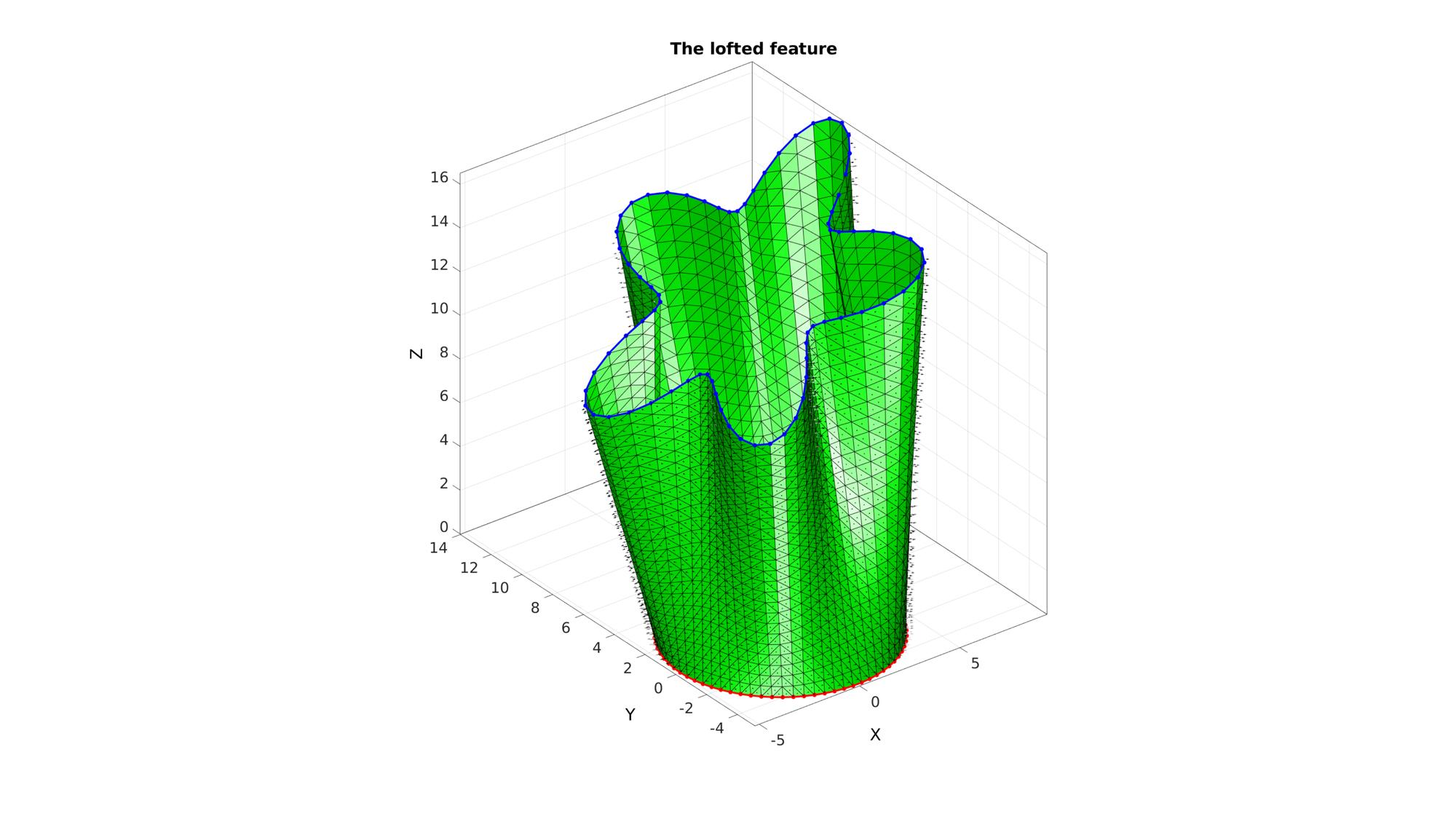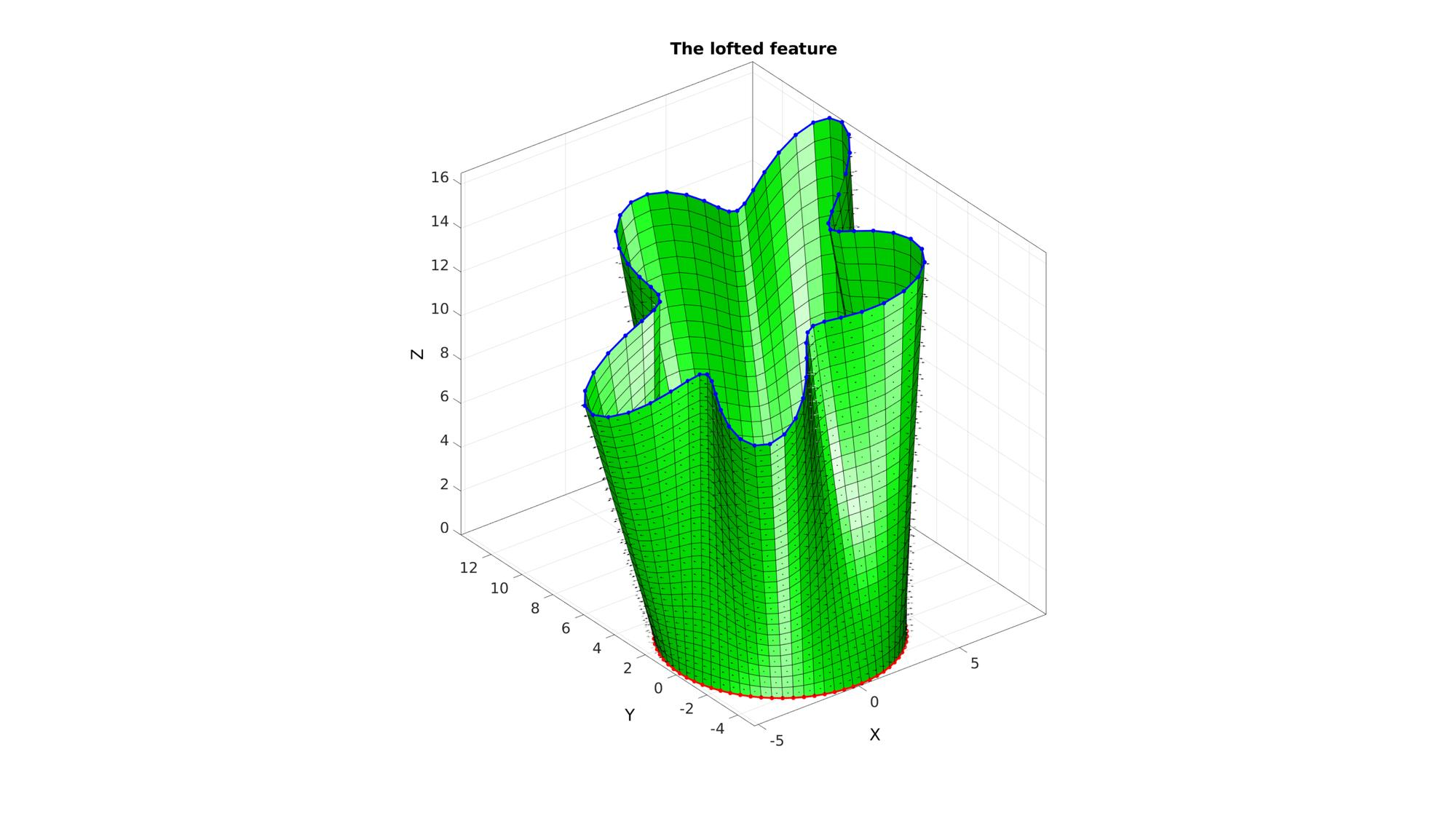# polyExtrude

Below is a demonstration of the features of the polyLoftLinear function

## Syntax

[F,V,indStart,indEnd]=polyLoftLinear(Vc_start,Vc_end,cPar);

## Description

The polyLoftLinear function can be used to loft a surface defined by a start and end polygon. This is similar to the loft feature in CAD software.

## Examples

```clear; close all; clc;
```

Plot settings

```figColor='w';
figColorDef='white';
fontSize=25;
markerSize1=25;
lineWidth1=3;
faceAlpha=0.5;
```

## Creating a loft feature

Sketching profile 1

```ns=75;
t=linspace(0,2*pi,ns);
t=t(1:end-1);
r=5;
x=r*cos(t);
y=r*sin(t);
z=zeros(size(x));
V_bottom=[x(:) y(:) z(:)];
```

Sketching profile 2

```t=linspace(0,2*pi,ns);
t=t(1:end-1);
r=6+2.*sin(5*t);
[x,y] = pol2cart(t,r);
V_top=[x(:) y(:) z(:)];
R=euler2DCM([0 -0.2*pi 0]);
V_top=(R*V_top')';
V_top(:,3)=V_top(:,3)+12;
V_top(:,2)=V_top(:,2)+6;
V_top(:,1)=V_top(:,1)+3;
```

Create loft cPar.numSteps=17;

```cPar.closeLoopOpt=1;
cPar.patchType='tri_slash';
[F,V]=polyLoftLinear(V_bottom,V_top,cPar);
```

Plotting results

```cFigure; hold on;
title('The lofted feature','FontSize',fontSize);

gpatch(F,V,'g','k',1);
patchNormPlot(F,V);

plotV(V_bottom,'r.-','lineWidth',lineWidth1,'MarkerSize',markerSize1);
plotV(V_top,'b.-','lineWidth',lineWidth1,'MarkerSize',markerSize1);

axisGeom(gca,fontSize);
drawnow;
```Create loft feature of a different type, also access start/end curve output.

```% cPar.numSteps=17;
cPar.closeLoopOpt=1;
[F,V,ind1,ind2]=polyLoftLinear(V_bottom,V_top,cPar);
```

Plotting results

```cFigure; hold on;
title('The lofted feature','FontSize',fontSize);

gpatch(F,V,'g','k',1);
patchNormPlot(F,V);
plotV(V(ind1,:),'r.-','lineWidth',lineWidth1,'MarkerSize',markerSize1);
plotV(V(ind2,:),'b.-','lineWidth',lineWidth1,'MarkerSize',markerSize1);

axisGeom(gca,fontSize);
drawnow;
```GIBBON www.gibboncode.org

Kevin Mattheus Moerman, [email protected]

GIBBON footer text

GIBBON: The Geometry and Image-based Bioengineering add-On. A toolbox for image segmentation, image-based modeling, meshing, and finite element analysis.

Copyright (C) 2019 Kevin Mattheus Moerman

This program is free software: you can redistribute it and/or modify it under the terms of the GNU General Public License as published by the Free Software Foundation, either version 3 of the License, or (at your option) any later version.

This program is distributed in the hope that it will be useful, but WITHOUT ANY WARRANTY; without even the implied warranty of MERCHANTABILITY or FITNESS FOR A PARTICULAR PURPOSE. See the GNU General Public License for more details.

You should have received a copy of the GNU General Public License along with this program. If not, see http://www.gnu.org/licenses/.